# Comparing Ratios

Comparing Ratios – To Compare two Ratios, we should follow the following steps : –

• Write both the Ratios as Fractions
• Convert both the Fractions into Like Fraction:-
– Find the L.C.M of denominator of both the Fractions
– Make the denominator of each fraction equal to their L.C.M.
• In case of Like fractions, the number whose numerator is greater is larger.

Comparing Ratios – Example 1

Compare the ratios ( 3 : 5 ) and ( 4 : 7 )

Explanation

We can write

( 3 : 5 ) = 3/5 and ( 4 : 7 ) = 4/7

Now, let us compare 3/5 and 4/7

LCM of 5 and 7 is 35

Making the denominator of each fraction equal to 35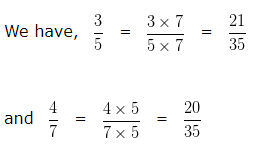In case of Like fractions, the number whose numerator is greater is larger.

Hence we can say 21/35 > 20/35

That is, 3/5 > 4/7

Hence, ( 3 : 5 ) > ( 4 : 7 )

Comparing Ratios – Example 2

Compare the ratios ( 1 : 5 ) and ( 2 : 7 )

Explanation

We can write

( 1 : 5 ) = 1/5 and ( 2 : 7 ) = 2/7

Now, let us compare 1/5 and 2/7

LCM of 5 and 7 is 35

Making the denominator of each fraction equal to 35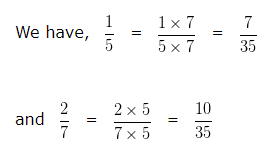In case of Like fractions, the number whose numerator is greater is larger.

Hence we can say 7/35 < 10/35

That is, 1/5 < 2/7

Hence, ( 1 : 5 ) < ( 2 : 7 )

Comparing Ratios – Example 3

Compare the ratios ( 7 : 4 ) and ( 6 : 8 )

Explanation

We can write

( 7 : 4 ) = 7/4 and ( 6 : 8 ) = 6/8

Now, let us compare 7/4 and 6/8

LCM of 4 and 8 is 8

Making the denominator of each fraction equal to 8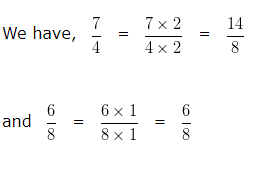In case of Like fractions, the number whose numerator is greater is larger.

Hence we can say 14/8 > 6/8

That is 7/4 > 6/8

Hence, ( 7 : 4 ) > ( 6 : 8 )

Comparing Ratios – Example 4

Compare the ratios ( 3 : 4 ) and ( 2 : 3 )

Explanation

We can write

( 3 : 4 ) = 3/4 and ( 2 : 3 ) = 2/3

Now, let us compare 3/4 and 2/3

LCM of 4 and 3 is 12

Making the denominator of each fraction equal to 12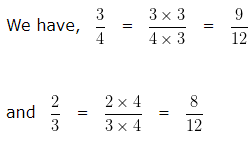In case of Like fractions, the number whose numerator is greater is larger.

Hence we can say 9/12 > 8/12

That is 3/4 > 2/3

Hence, ( 3 : 4 ) > ( 2 : 3 )

Comparing Ratios – Example 5

Compare the ratios ( 1 : 5 ) and ( 1 : 4 )

Explanation

We can write

( 1 : 5 ) = 1/5 and ( 1 : 4 ) = 1/4

Now, let us compare 1/5 and 1/4

LCM of 5 and 4 is 20

Making the denominator of each fraction equal to 20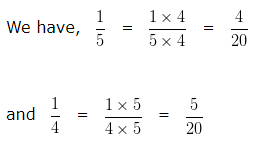In case of Like fractions, the number whose numerator is greater is larger.

Hence we can say 4/20 < 5/20

That is, 1/5 < 1/4

Hence, ( 1 : 5 ) < ( 1 : 4 )